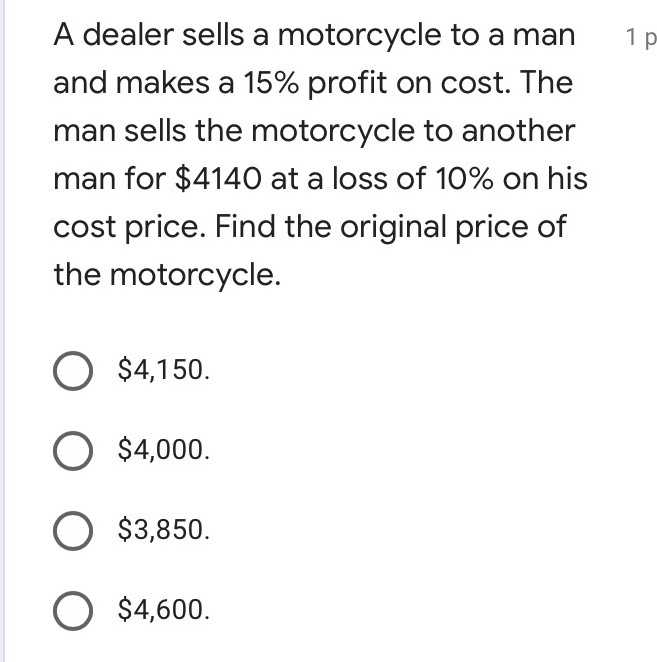### ¿Todavía tienes preguntas de matemáticas?

Pregunte a nuestros tutores expertos
Algebra
PreguntaA dealer sells a motorcycle to a man and makes a $$15 \%$$ profit on cost. The man sells the motorcycle to another man for $$\ 4140$$ at a loss of $$10 \%$$ on his cost price. Find the original price of the motorcycle.

$$\ 4,150 .$$

$$\ 4,000 .$$

$$\ 3,850 .$$

$$\ 4,600 .$$

$$\ 4,000$$